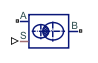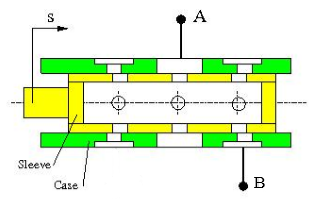# Variable Overlapping Orifice (IL)

Orifice created by open segments with variable overlap in an isothermal liquid system

Since R2020a

•Libraries:
Simscape / Fluids / Isothermal Liquid / Valves & Orifices / Orifices

## Description

The Variable Overlapping Orifice (IL) block models flow through round holes with varying overlapping areas, such as a moving sleeve within a fixed case. The overlapping holes can have different diameters, but additional holes along the spool or sleeve have the same diameter.### Overlapping Area

The flow rate depends on the variable open area created by overlapping holes in the sleeve and casing. This instantaneous opening area is calculated as:

`$\begin{array}{l}{A}_{overlap}={r}^{2}\left[{\mathrm{cos}}^{-1}\left(\frac{{C}^{2}+{r}^{2}-{R}^{2}}{2Cr}\right)-\left(\frac{{C}^{2}+{r}^{2}-{R}^{2}}{2Cr}\right)\sqrt{1-{\left(\frac{{C}^{2}+{r}^{2}-{R}^{2}}{2Cr}\right)}^{2}}\right]\\ +{R}^{2}\left[{\mathrm{cos}}^{-1}\left(\frac{{C}^{2}-{r}^{2}+{R}^{2}}{2CR}\right)-\left(\frac{{C}^{2}-{r}^{2}+{R}^{2}}{2CR}\right)\sqrt{1-{\left(\frac{{C}^{2}-{r}^{2}+{R}^{2}}{2CR}\right)}^{2}}\right],\end{array}$`

where:

• r is the diameter of the smaller hole.

• R is the diameter of the larger hole.

• C is the absolute distance between the hole centers, calculated from the physical signal at port S, the instantaneous sleeve position, and the Sleeve position when holes are concentric, S0: $C=|S-{S}_{0}|.$

If the holes on the sleeve and casing have the same diameter, the overlap area becomes:

`${A}_{overlap}=2{r}^{2}\left[{\mathrm{cos}}^{-1}\left(\frac{C}{2r}\right)-\left(\frac{C}{2r}\right)\sqrt{1-{\left(\frac{C}{2r}\right)}^{2}}\right].$`

You can maintain numerical robustness in your simulation by adjusting the block at the nearest and farthest points between hole centers. The block applies a smoothing function to the normalized hole center distance,

`$\stackrel{^}{C}=\frac{\left(C-R+r\right)}{\left(2r\right)}.$`

The function smoothly saturates the normalized hole center distance between `0` and `1`.

### Mass Flow Rate Equation

The flow through an orifice pair is calculated from the pressure-area relationship:

`$\stackrel{˙}{m}=\frac{{C}_{d}{A}_{orifice}\sqrt{2\overline{\rho }}}{\sqrt{P{R}_{loss}\left(1-{\left(\frac{{A}_{orifice}}{{A}_{port}}\right)}^{2}\right)}}\frac{\Delta p}{{\left[\Delta {p}^{2}+\Delta {p}_{crit}^{2}\right]}^{1/4}},$`

where:

• Cd is the Discharge coefficient.

• Aorifice is the area open to flow, ${A}_{orifice}={A}_{overlap}+{A}_{leak}.$

• A is the Cross-sectional area at ports A and B.

• $\overline{\rho }$ is the average fluid density.

Pressure loss describes the reduction of pressure in the valve due to a decrease in area. The pressure loss term, PRloss is calculated as:

`$P{R}_{loss}=\frac{\sqrt{1-{\left(\frac{{A}_{overlapping}}{{A}_{port}}\right)}^{2}\left(1-{C}_{d}^{2}\right)}-{C}_{d}\frac{{A}_{overlapping}}{{A}_{port}}}{\sqrt{1-{\left(\frac{{A}_{overlapping}}{{A}_{port}}\right)}^{2}\left(1-{C}_{d}^{2}\right)}+{C}_{d}\frac{{A}_{overlapping}}{{A}_{port}}}.$`

Pressure recovery describes the positive pressure change in the valve due to an increase in area. If you do not wish to capture this increase in pressure, set Pressure recovery to `Off`. In this case, PRloss is 1.

The critical pressure difference, Δpcrit, is the pressure differential associated with the Critical Reynolds number, Recrit, the flow regime transition point between laminar and turbulent flow:

`$\Delta {p}_{crit}=\frac{\pi \overline{\rho }}{8{A}_{overlapping}}{\left(\frac{\nu {\mathrm{Re}}_{crit}}{{C}_{d}}\right)}^{2}.$`

## Ports

### Conserving

expand all

Entry or exit point to the orifice.

Entry or exit point to the orifice.

### Input

expand all

Sleeve position with respect to the case, in m.

## Parameters

expand all

Diameter of the hole in the moving element.

Diameter of hole in the fixed element.

Moving element position when hole centers are aligned. This parameter is used to the determine instantaneous overlap of the moving and stationary holes during simulation by comparison with the signal received at port S.

Number of overlapping orifices, in pairs. The sleeve-case pairs do not need to be aligned in the physical hardware being modeled.

Sum of all gaps when the valve is in the fully closed position. Any area smaller than this value is maintained at the specified leakage area. This contributes to numerical stability by maintaining continuity in the flow.

Areas at the entry and exit ports A and B, which are used in the pressure-flow rate equation that determines the mass flow rate through the orifice.

Correction factor that accounts for discharge losses in theoretical flows.

Upper Reynolds number limit for laminar flow through the orifice.

Continuous smoothing factor that introduces a layer of gradual change to the flow response when the valve is in near-open or near-closed positions. Set this value to a nonzero value less than one to increase the stability of your simulation in these regimes.

Whether to account for pressure increase when fluid flows from a region of smaller cross-sectional area to a region of larger cross-sectional area.

## Version History

Introduced in R2020a